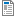# 17ABBMEC - Mechanics

Code Completion Credits Range Language
17ABBMEC Z,ZK 4 2P+2L English
Lecturer:
Patrik Kutílek
Tutor:
Patrik Kutílek
Supervisor:
Department of Natural Sciences
Synopsis:

Cross-section characteristics, body stress state ( Cauchy, geometry, compatibility and physical equations), linear elasticity theory, reaction, beam bending, normal and tangential stresses, deformation, torsion influence.

Requirements:

Credit condition - 50% presence, laboratory works and submit reports from laboratory works. Take the final exam and receive an mark A-F. The minimum to pass the exam is fifty percent of the six questions.

The questions in the test are from the following areas:

Cross-section characteristics, body stress state ( Cauchy, geometry, compatibility and physical equations), linear elasticity theory, reaction, beam bending, normal and tangential stresses, deformation, torsion influence.

Syllabus of lectures:

1. Basic physics equations, Newton's laws, statics and dynamics.

2. Force and torque effects and operations with them.

3. The balance, center of area and mass, equations of equilibrium.

4. Reactions, static systems, movement restrictions, reaction solutions.

5. Torque,moment of inertia, rotation.

6. Angular momentum, conservation of angular momentum.

7. Surface moment of inertia, principal moments of inertia, polar moment, Mohr's circle, ellipse of inertia.

8. Static analysis of beam, internal effects, kinematic method, statically indeterminate problems.

9. Mechanical properties of materials, testing of mechanical properties, stress and strain, Hooke's law.

10. Stress of the material, uniaxial and biaxial stress state, bending.

11. Torsion stress, polar moment.

12. Combined stress,

13. Critical load, the stability of rods.

Syllabus of tutorials:

1. Basic physics equations, Newton's laws, statics and dynamics.

2. Force and torque effects and operations with them.

3. The balance, center of area and mass, equations of equilibrium.

4. Reactions, static systems, movement restrictions, reaction solutions.

5. Torque,moment of inertia, rotation.

6. Angular momentum, conservation of angular momentum.

7. Surface moment of inertia, principal moments of inertia, polar moment, Mohr's circle, ellipse of inertia.

8. Static analysis of beam, internal effects, kinematic method, statically indeterminate problems.

9. Mechanical properties of materials, testing of mechanical properties, stress and strain, Hooke's law.

10. Stress of the material, uniaxial and biaxial stress state, bending.

11. Torsion stress, polar moment.

12. Combined stress,

13. Critical load, the stability of rods.

Study Objective:

Cross-section characteristics, body stress state ( Cauchy, geometry, compatibility and physical equations), linear elasticity theory, reaction, beam bending, normal and tangential stresses, deformation, torsion influence.

Study materials:

1. Ranakrishna S., Huang Z., Kumar V., Batchelor W., Mayer J.: An Introduction to Biocomposites.

2. Freitas R.,A.: Nanomedicine - Biocompatibility.

3. Brown B., Smallwood R., Barber D.: Medical physics and biomedical engineering.

4. Kutílek, P., Žižka, A. Selected Chapters from Experimental Biomechanics. 1. Vydání.Praha: Česká technika - nakladatelství ČVUT, 2012, 165 s., ISBN 978-80-01-05114-6.

Note:
The course is a part of the following study plans:17ABBMEC_20170501_151822_e3620129d356f6ea021ce23c87f025b9.pdf125.84 KBSchedule 17/1854 KBSchedule 18/19104.8 KBSchedule 19/2080.71 KBSchedule 20/2180.71 KB